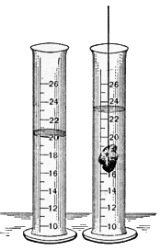xDisplacement (fluid)Overview

In fluid mechanics
Fluid mechanics
Fluid mechanics is the study of fluids and the forces on them. Fluid mechanics can be divided into fluid statics, the study of fluids at rest; fluid kinematics, the study of fluids in motion; and fluid dynamics, the study of the effect of forces on fluid motion...

, displacement occurs when an object is immersed in a fluid
Fluid
In physics, a fluid is a substance that continually deforms under an applied shear stress. Fluids are a subset of the phases of matter and include liquids, gases, plasmas and, to some extent, plastic solids....

, pushing it out of the way and taking its place. The volume of the fluid displaced can then be measured, as in the illustration, and from this the volume of the immersed object can be deduced (the volume of the immersed object will be exactly equal to the volume of the displaced fluid).

An object that sinks displaces an amount of fluid equal to the object's volume.Encyclopedia
In fluid mechanics
Fluid mechanics
Fluid mechanics is the study of fluids and the forces on them. Fluid mechanics can be divided into fluid statics, the study of fluids at rest; fluid kinematics, the study of fluids in motion; and fluid dynamics, the study of the effect of forces on fluid motion...

, displacement occurs when an object is immersed in a fluid
Fluid
In physics, a fluid is a substance that continually deforms under an applied shear stress. Fluids are a subset of the phases of matter and include liquids, gases, plasmas and, to some extent, plastic solids....

, pushing it out of the way and taking its place. The volume of the fluid displaced can then be measured, as in the illustration, and from this the volume of the immersed object can be deduced (the volume of the immersed object will be exactly equal to the volume of the displaced fluid).

An object that sinks displaces an amount of fluid equal to the object's volume. Thus buoyancy
Buoyancy
In physics, buoyancy is a force exerted by a fluid that opposes an object's weight. In a column of fluid, pressure increases with depth as a result of the weight of the overlying fluid. Thus a column of fluid, or an object submerged in the fluid, experiences greater pressure at the bottom of the...

is expressed through Archimedes' principle
Archimedes' principle
Archimedes' principle relates buoyancy to displacement. It is named after its discoverer, Archimedes of Syracuse.-Principle:Archimedes' treatise On floating bodies, proposition 5, states that...

, which states that the weight
Weight
In science and engineering, the weight of an object is the force on the object due to gravity. Its magnitude , often denoted by an italic letter W, is the product of the mass m of the object and the magnitude of the local gravitational acceleration g; thus:...

of the object is reduced by its volume multiplied by the density
Density
The mass density or density of a material is defined as its mass per unit volume. The symbol most often used for density is ρ . In some cases , density is also defined as its weight per unit volume; although, this quantity is more properly called specific weight...

of the fluid. If the weight of the object is less than this displaced quantity, the object floats; if more, it sinks. The amount of fluid displaced is directly related (via Archimedes' Principle) to its weight.

In the case of an object that sinks (is totally submerged), the volume of the object is displaced. In the case of an object that floats, the amount of fluid displaced will be equal in weight to the displacing object.

## Applications of displacement

Commonly the water displacement, this method can be used to measure
Measurement
Measurement is the process or the result of determining the ratio of a physical quantity, such as a length, time, temperature etc., to a unit of measurement, such as the metre, second or degree Celsius...

the volume of a solid object, even if its form is not regular. Several methods of such measuring exist. In one case the increase of water level is registered as the object is immersed into the water. In the second case the object is immersed into a vessel full of water, causing it to overflow. Then the overflown water is collected and its volume measured. In the third case the object is suspended under the surface of the water and the increase of weight of the vessel is measured. It is equivalent to the weight of the amount of water that has the volume equal to the one of the suspended object.

The weight of an object or substance can be measured by floating a sufficiently buoyant receptacle in the cylinder and noting the water level. After placing the object or substance in the receptacle, the difference in weight of the water level volumes will equal the weight of the object.It is a method